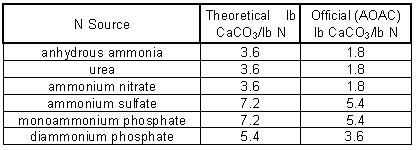## Competency Area 5: Soil pH and Liming

### PO 37. Describe the long-term change in soil pH from applying N.

Nitrification or the conversion of ammonium N to nitrate N produces acidity:

2 NH4+ + 4 O2 → 2 NO3- + 4 H+ + H2O

2 H+ are produced for every N in the ammonium-N form (NH4+).  This reaction occurs regardless of the source of the NH4+.  This acidity is often the largest single source of acidity in fertilized agricultural soils.

The net amount of acidity created when N fertilizer is applied depends on other reactions that occur with the fertilizer.  The acidity created by different fertilizer materials is summarized below, as is the lb CaCO3 required to neutralize the acid.This information can be used to calculate the amount of lime required to neutralize a given application of fertilizer. For example:

• Find the CaCO3 equivalent (lime requirement) of 150 lb N/acre applied as urea: 150 lb N x 1.8 lb CaCO3/lb N = 270 lb lime requirement
• Find the CaCO3 equivalent of 150 lb N/acre applied as ammonium sulfate: 150 lb N x 5.4 lb CaCO3/lb N = 810 lb lime requirement.

The following reactions explain why there are differences in the amount of acidity per pound of N for the different N fertilizer materials.

• Ammonia: NH3 + H2O → NH4+ + OH-
• The reaction produces 1 OH- per N.  This OH- neutralizes one of the two H+ that will be produced by nitrification – ½ of the acidity is neutralized.
• Urea: NH2CONH2 + H2O → 2NH3 + 2H2O → 2NH4+ + 2OH-
• The reaction produces 1 OH- per N.  This OH- neutralizes one of the two H+ that will be produced by nitrification – ½ of the acidity is neutralized.
• Ammonium Nitrate: NH4NO3
• No OH- is produced when this fertilizer reacts.  As only one N is NH4+ (the other is already NO3- and does not need conversion), only one H+ is formed per N.
• Ammonium Sulfate: (NH4)2SO3
• No OH- is produced when this fertilizer reacts; therefore, all the acidity produced by nitrification remains and none is neutralized.
• Monoammonium Phosphate (MAP): NH4H2PO4
• No OH- is produced when this fertilizer reacts; therefore, all the acidity produced by nitrification remains and none is neutralized.
• Diammonium Phosphate (DAP): (NH4)2HPO4 + H2O → 2NH4 + HPO42- + H2O → H2PO4- + OH
• The reaction produces 1 OH- per 2N.  This OH- neutralizes one of the four H+ that will be produced by nitrification – ¼ of the acidity is neutralized.